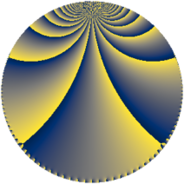# Properties

 Label 252.4.oLevel $252$ Weight $4$ Character orbit 252.o Rep. character $\chi_{252}(95,\cdot)$ Character field $\Q(\zeta_{6})$ Dimension $280$ Sturm bound $192$

# Learn more

## Defining parameters

 Level: $$N$$ $$=$$ $$252 = 2^{2} \cdot 3^{2} \cdot 7$$ Weight: $$k$$ $$=$$ $$4$$ Character orbit: $$[\chi]$$ $$=$$ 252.o (of order $$6$$ and degree $$2$$) Character conductor: $$\operatorname{cond}(\chi)$$ $$=$$ $$252$$ Character field: $$\Q(\zeta_{6})$$ Sturm bound: $$192$$

## Dimensions

The following table gives the dimensions of various subspaces of $$M_{4}(252, [\chi])$$.

Total New Old
Modular forms 296 296 0
Cusp forms 280 280 0
Eisenstein series 16 16 0

## Trace form

 $$280q - 3q^{2} + q^{4} - 12q^{6} - 2q^{9} + O(q^{10})$$ $$280q - 3q^{2} + q^{4} - 12q^{6} - 2q^{9} + 14q^{10} - 165q^{12} - 4q^{13} - 3q^{14} + q^{16} + 53q^{18} - 6q^{20} + 240q^{21} - 18q^{22} - 278q^{24} - 6204q^{25} - 24q^{26} + 60q^{28} - 96q^{29} - 494q^{30} - 693q^{32} - 218q^{33} - 10q^{34} - 658q^{36} - 4q^{37} + 248q^{40} - 12q^{41} - 313q^{42} + 771q^{44} + 1218q^{45} - 18q^{46} + 509q^{48} - 2q^{49} + 369q^{50} + 254q^{52} + 68q^{54} + 942q^{56} + 308q^{57} - 34q^{58} + 464q^{60} + 2q^{61} - 8q^{64} - 702q^{65} - 435q^{66} - 2118q^{69} + 858q^{70} + 1565q^{72} - 4q^{73} - 66q^{76} + 978q^{77} - 821q^{78} - 3297q^{80} - 1390q^{81} - 10q^{82} + 17q^{84} - 254q^{85} - 1026q^{88} - 3180q^{89} + 1199q^{90} + 3984q^{92} - 222q^{93} + 33q^{94} + 5404q^{96} - 4q^{97} + 4515q^{98} + O(q^{100})$$

## Decomposition of $$S_{4}^{\mathrm{new}}(252, [\chi])$$ into newform subspaces

The newforms in this space have not yet been added to the LMFDB.